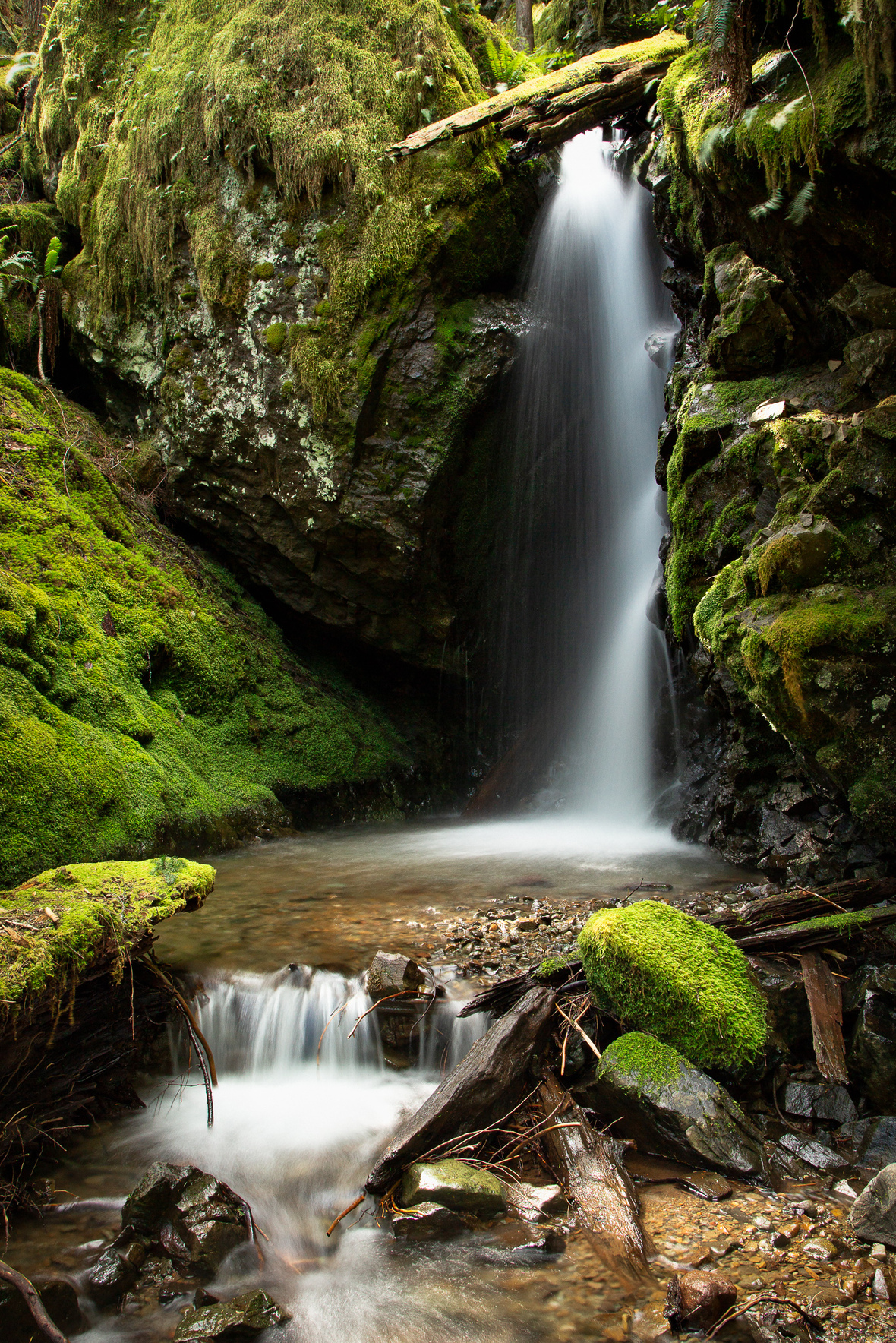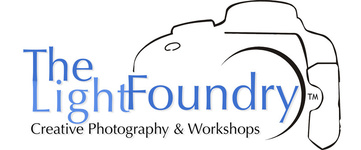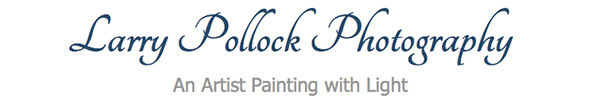## June 2018 v5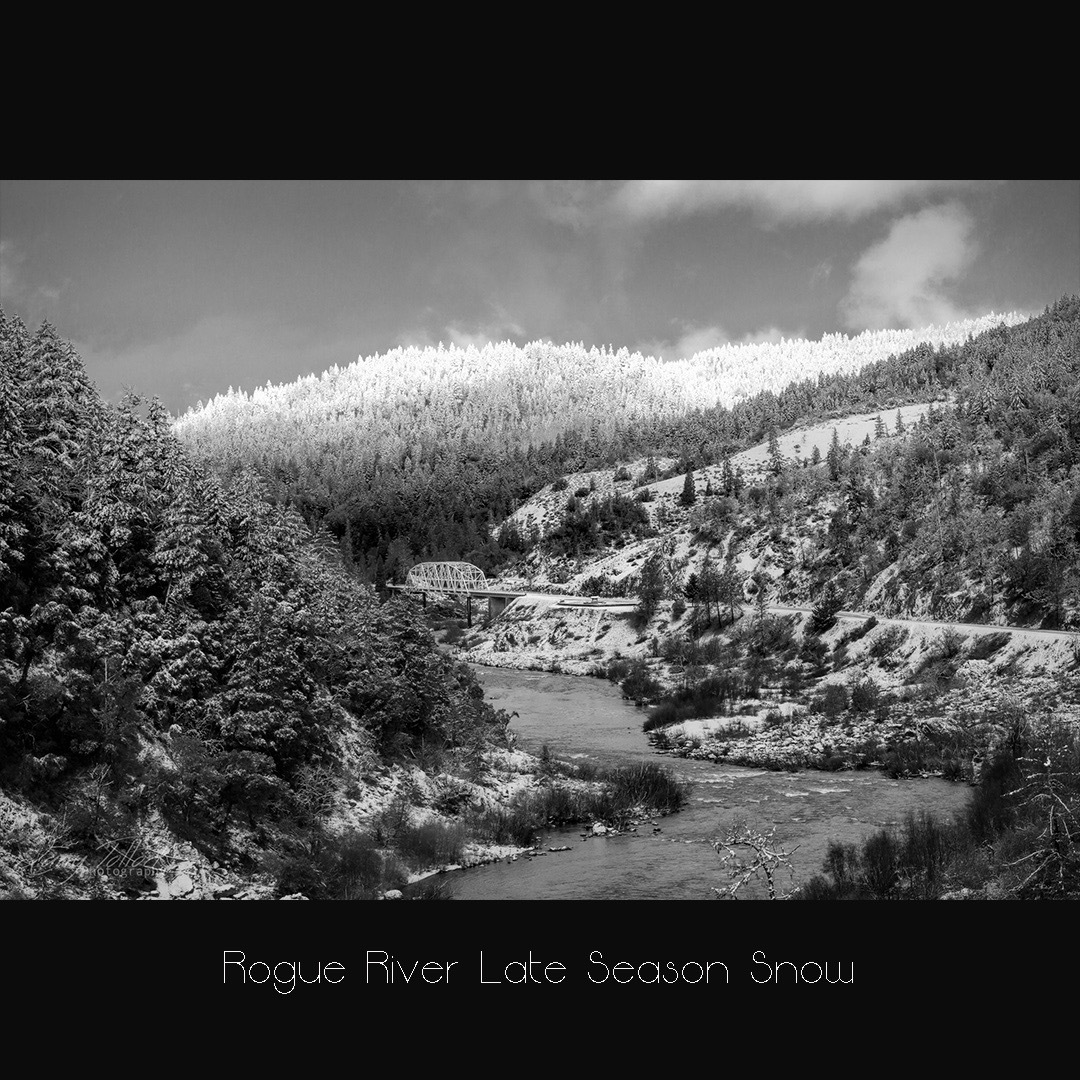Snow fell in Merlin and I dashed down to the Hellgate Overlook on the Rogue River. 6 inches of fresh untouched snow was all mine.

 table div table+table+table+table+table div table{width:100%;padding:0}table div table+table+table+table+table div table img{width:96.23%;padding:0;float:none}table div table+table+table+table+table div table td{width:100%;padding:0 1.88% 18px}/* styles */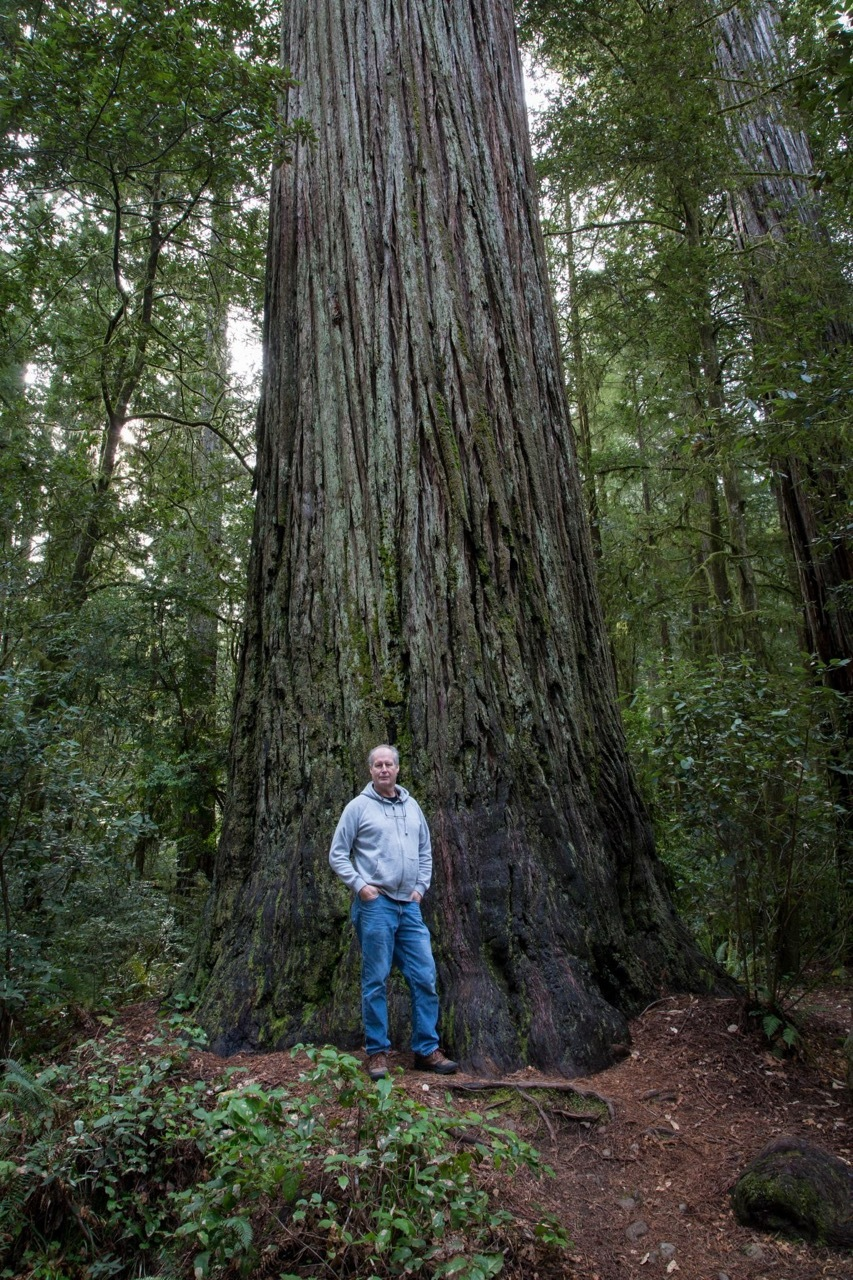Taking some time to go visit the Redwoods, I camped at Jedediah Smith State Park. A 2 hour drive down the Smith River and just outside of Crescent City. I went a few weeks before Memorial Weekend to avoid the summer crowds. Driving up and down the coast, hiking out to points near Klamath and hikes in the redwood groves, the weather was perfect. I only wish I had stayed longer than 3 days. Driving back to Merlin I stopped and visited the Oregon Caves. I will return to the coast as it is virtually in my backyard.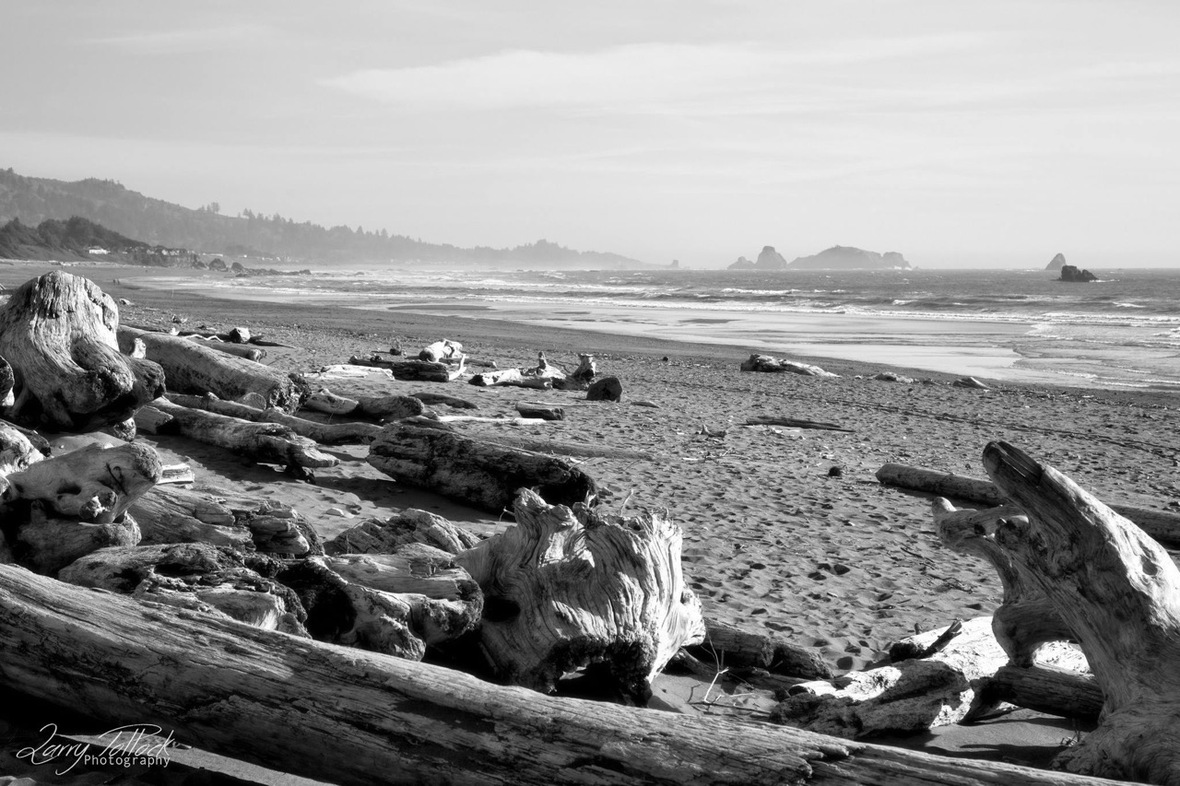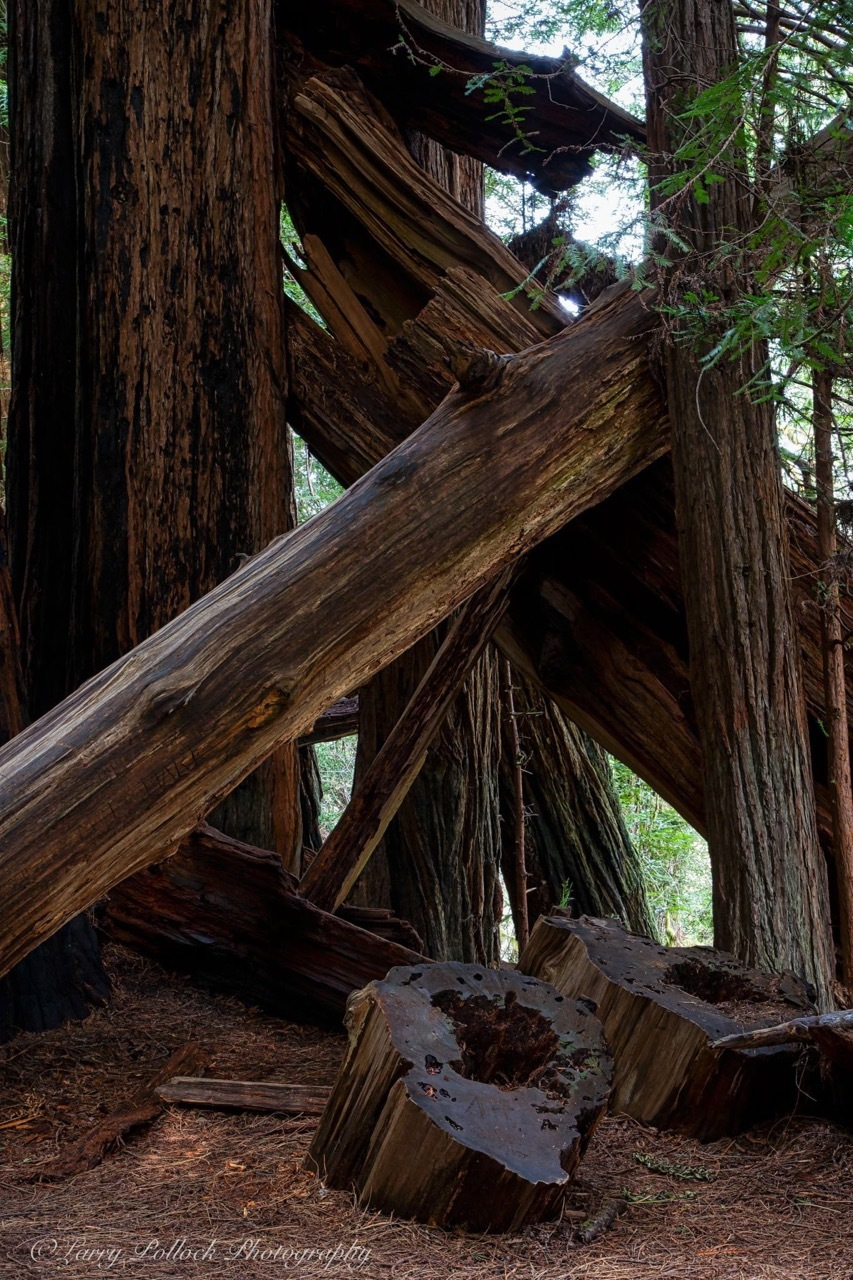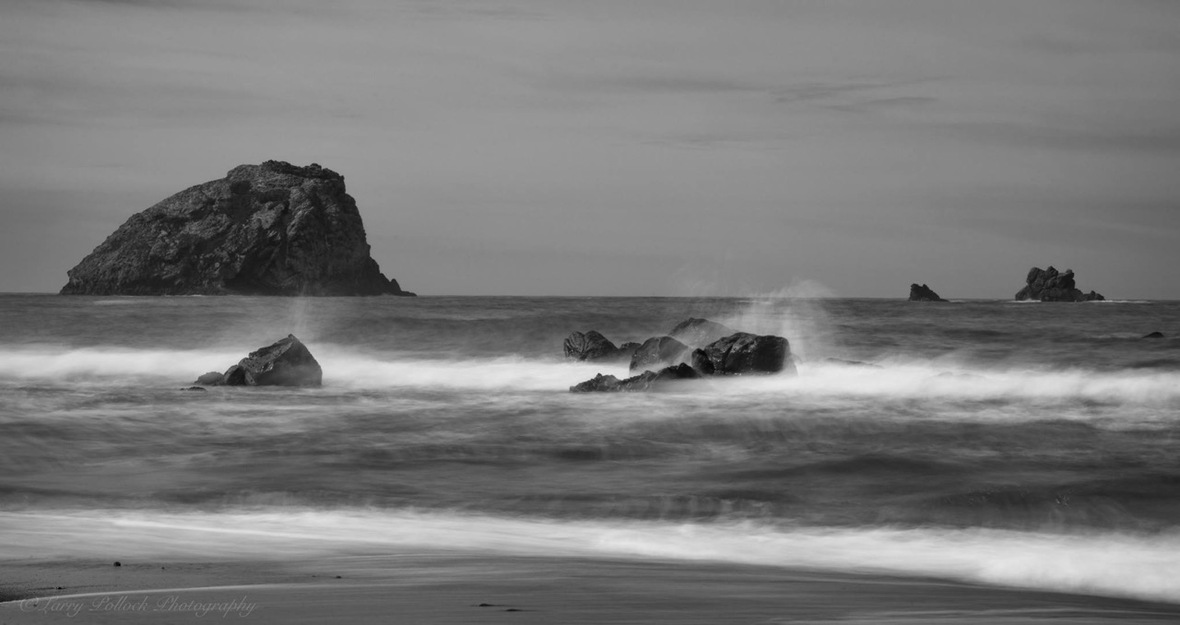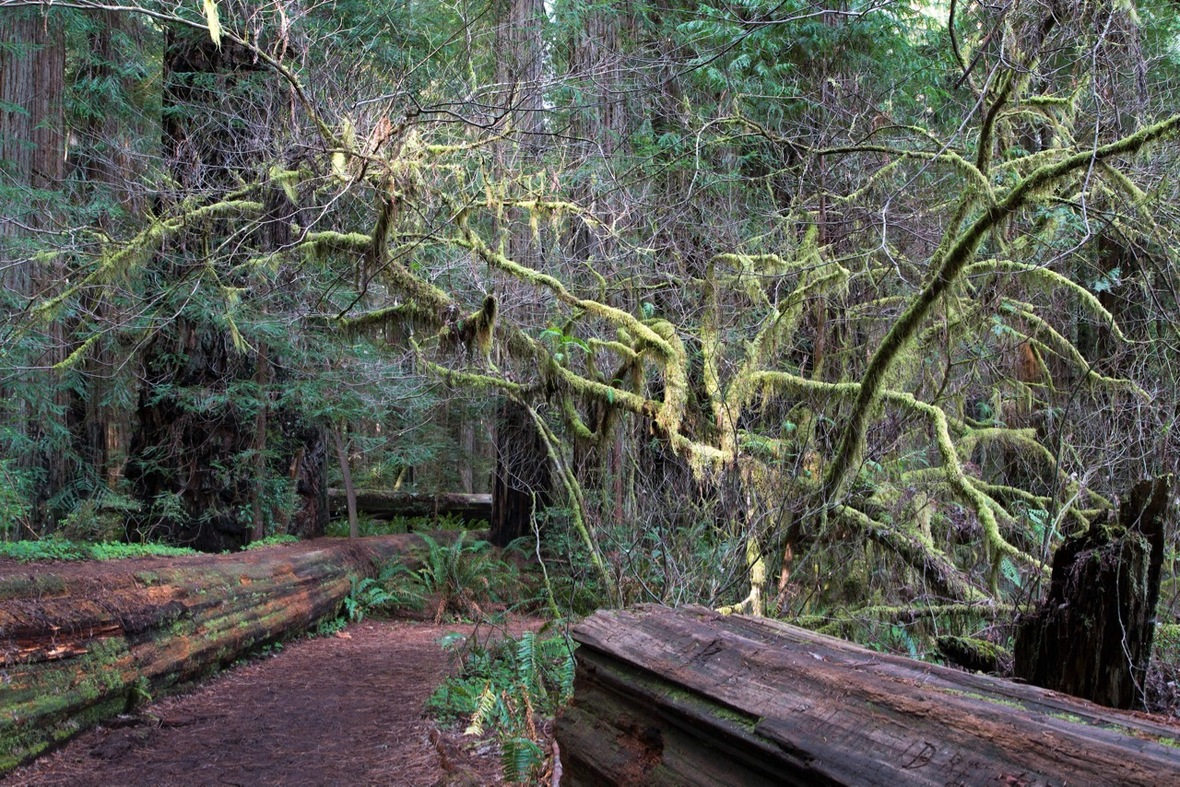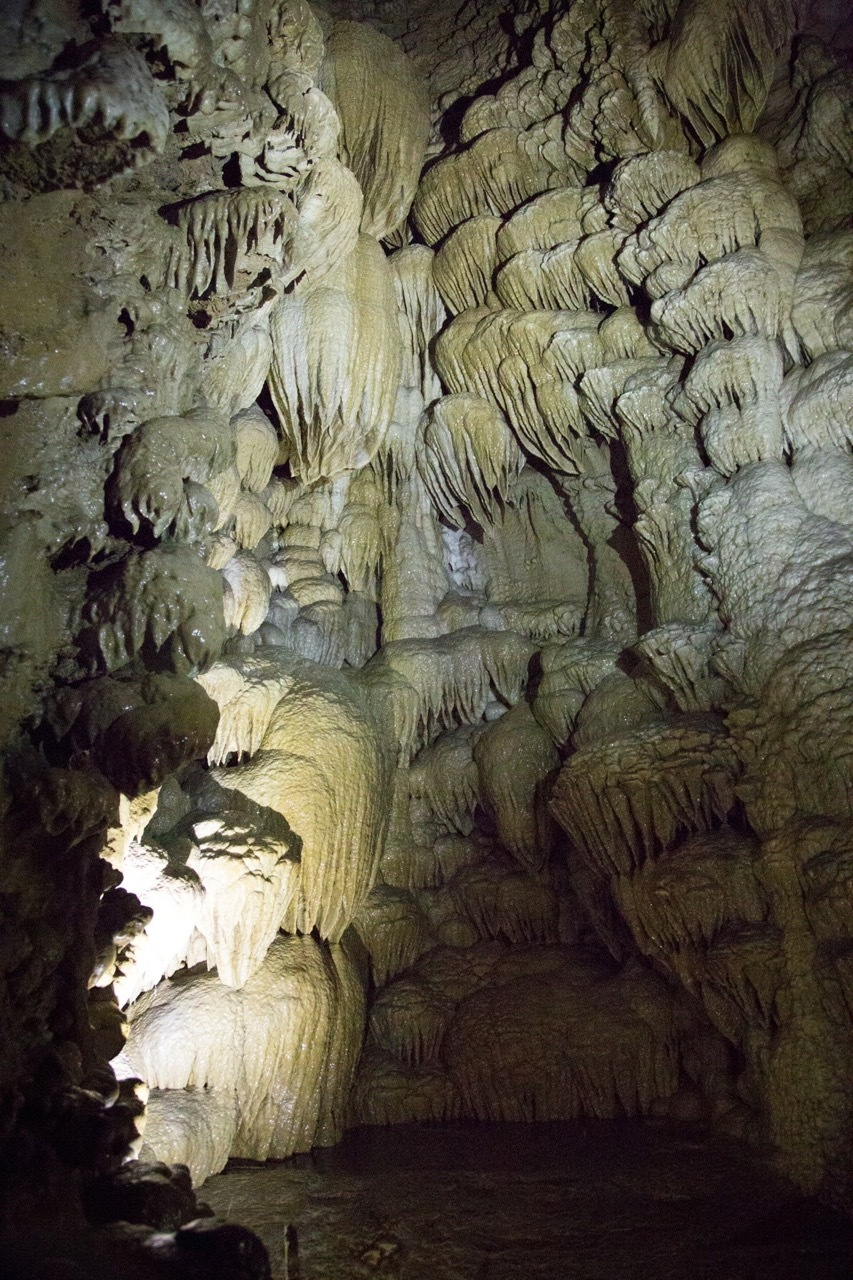table div table+table+table+table+table+table+table+table+table+table+table+table div table{width:100%;padding:0}table div table+table+table+table+table+table+table+table+table+table+table+table div table img{width:96.23%;padding:0;float:none}table div table+table+table+table+table+table+table+table+table+table+table+table div table td{width:100%;padding:0 1.88% 18px}/* styles */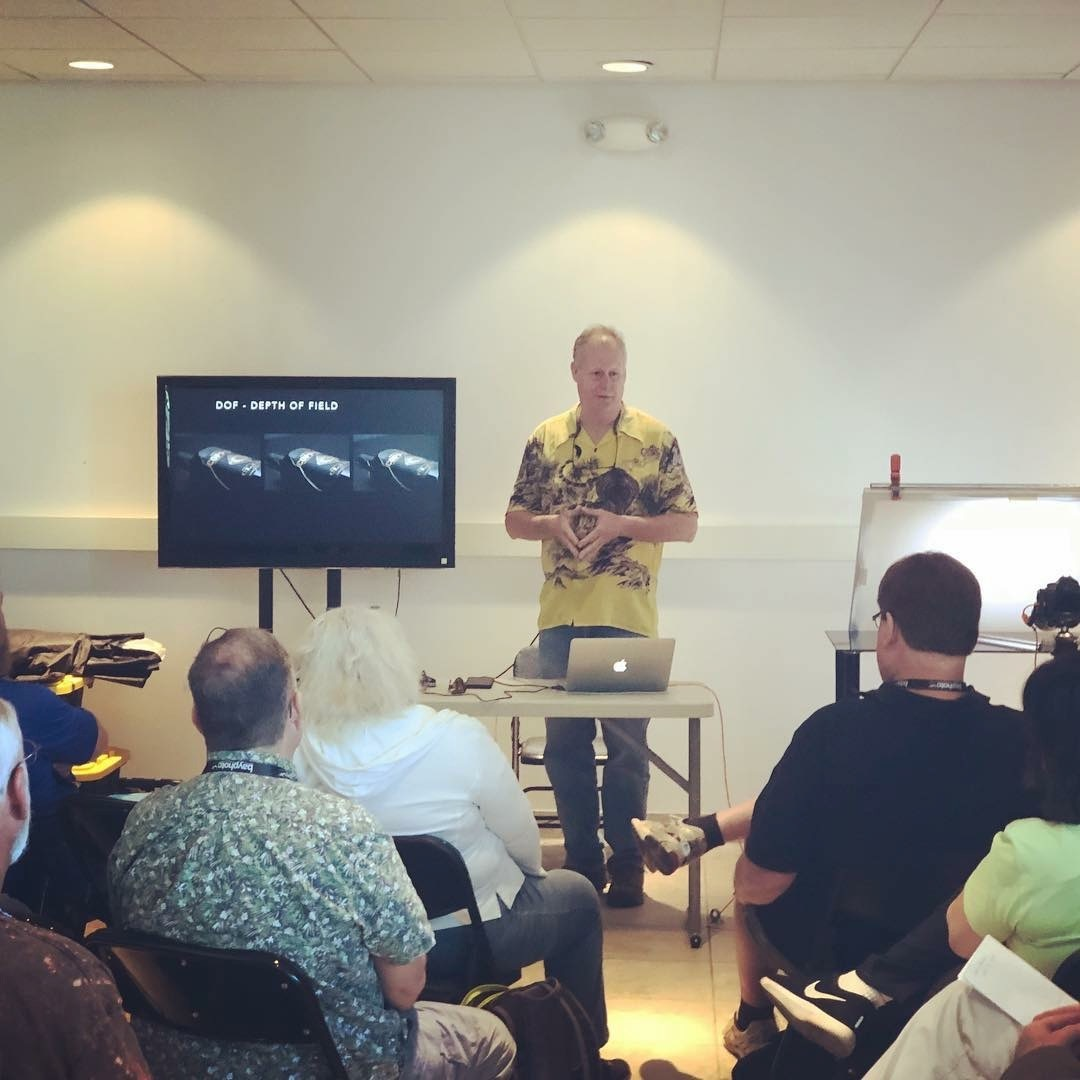Being asked to return and speak at the Capture Photo Con in Phoenix, I planned a two week road trip. Arriving in Santa Cruz CA, I stayed with several friends in Aptos. Visiting the old home town on some family business as well as a chance to get together with my old surfing buddies and their wives was something I had to do on the trip. This worked out perfectly and fun was had by all. A short trip to the beach and some photos of the cement ship, the Palo Alto, that we played in as kids. It is now broken and unsafe.

I took two days to drive to Phoenix. Palm Desert was the first stop after braving the LA traffic over the Grapevine and across to the east from Burbank. Arriving in Phoenix, I stayed with my friend Patrick and his wife. Always great as we talk food, wine and photography. He even developed some old film in a solution of instant coffee and vitamin C, with a second solution of washing soda. Hey, it works!

The Capture Photo Con is a convention on all things photography. Not only do I get to teach several classes and do studio lighting demos with my favorite models, I also get to attend other classes and demos from other wonderful photographers. Going out to dinner and hanging out with friends.

I taught a class on a technique called Focus Stacking for complete in focus image using Macro Lenses or shooting Landscape images. The second class was on product photography and the basics of lighting and making a beer glass and beer look cold, wet and frosty. Both classes had more sign ups than seats. We made room for all in these overbooked classes.

Down on the main floor with all the vendors I did Film Noir style lighting demos in the full daylight of a basket ball court with windows and skylights! I had no idea what model were scheduled for that time. To my surprise 3 of my favorites showed up ready to play and shoot in the studio lights. Aurora O’Brien, Kim Wright and Lindsey Lockwood. Fun was had by all and it was a great reunion. I’ll let the photos tell the story

 table.module-13{width:0%;padding:0}table div table+table+table+table+table+table+table+table+table+table+table+table+table+table div table{width:0%;float:none;margin-left:auto;margin-right:auto;padding:0}table div table+table+table+table+table+table+table+table+table+table+table+table+table+table div table a{border:0 none;text-decoration:none}table div table+table+table+table+table+table+table+table+table+table+table+table+table+table div table img{width:100%!important;border:0 none;text-decoration:none}table div table+table+table+table+table+table+table+table+table+table+table+table+table+table div table td{width:100%;padding:0}/* styles */
 table.module-14{width:0%;padding:0}table div table+table+table+table+table+table+table+table+table+table+table+table+table+table+table div table{width:0%;float:none;margin-left:auto;margin-right:auto;padding:0}table div table+table+table+table+table+table+table+table+table+table+table+table+table+table+table div table a{border:0 none;text-decoration:none}table div table+table+table+table+table+table+table+table+table+table+table+table+table+table+table div table img{width:100%!important;border:0 none;text-decoration:none}table div table+table+table+table+table+table+table+table+table+table+table+table+table+table+table div table td{width:100%;padding:0}/* styles */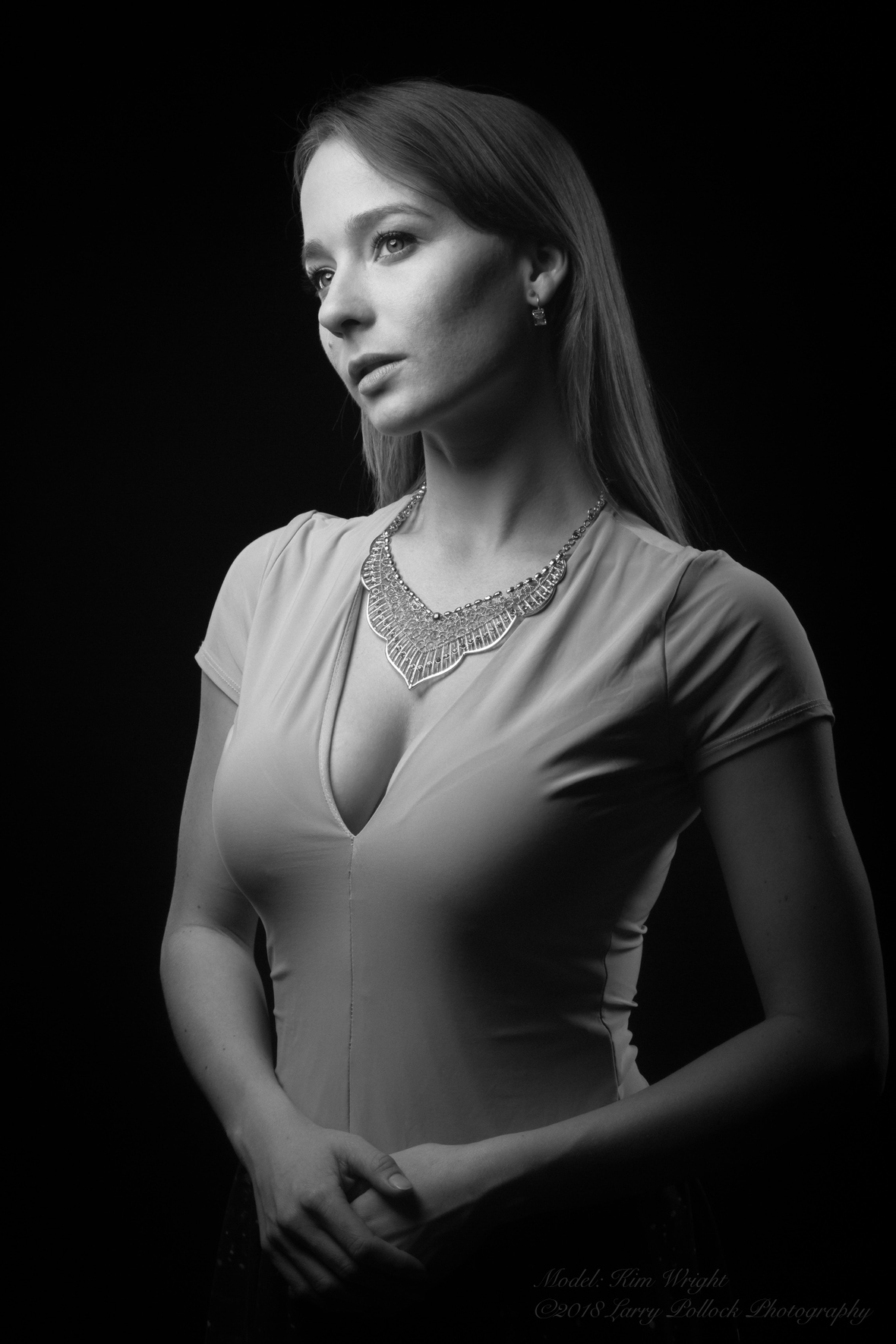table.module-16{width:0%;padding:0}table div table+table+table+table+table+table+table+table+table+table+table+table+table+table+table+table+table div table{width:0%;float:none;margin-left:auto;margin-right:auto;padding:0}table div table+table+table+table+table+table+table+table+table+table+table+table+table+table+table+table+table div table a{border:0 none;text-decoration:none}table div table+table+table+table+table+table+table+table+table+table+table+table+table+table+table+table+table div table img{width:100%!important;border:0 none;text-decoration:none}table div table+table+table+table+table+table+table+table+table+table+table+table+table+table+table+table+table div table td{width:100%;padding:0}/* styles */
 table div table+table+table+table+table+table+table+table+table+table+table+table+table+table+table+table+table+table div table{width:100%;padding:0}table div table+table+table+table+table+table+table+table+table+table+table+table+table+table+table+table+table+table div table img{width:96.23%;padding:0;float:none}table div table+table+table+table+table+table+table+table+table+table+table+table+table+table+table+table+table+table div table td{width:100%;padding:0 1.88% 18px}/* styles */table div table+table+table+table+table+table+table+table+table+table+table+table+table+table+table+table+table+table+table+table+table div table{width:100%;padding:0}table div table+table+table+table+table+table+table+table+table+table+table+table+table+table+table+table+table+table+table+table+table div table img{width:96.23%;padding:0;float:none}table div table+table+table+table+table+table+table+table+table+table+table+table+table+table+table+table+table+table+table+table+table div table td{width:100%;padding:0 1.88% 18px}/* styles */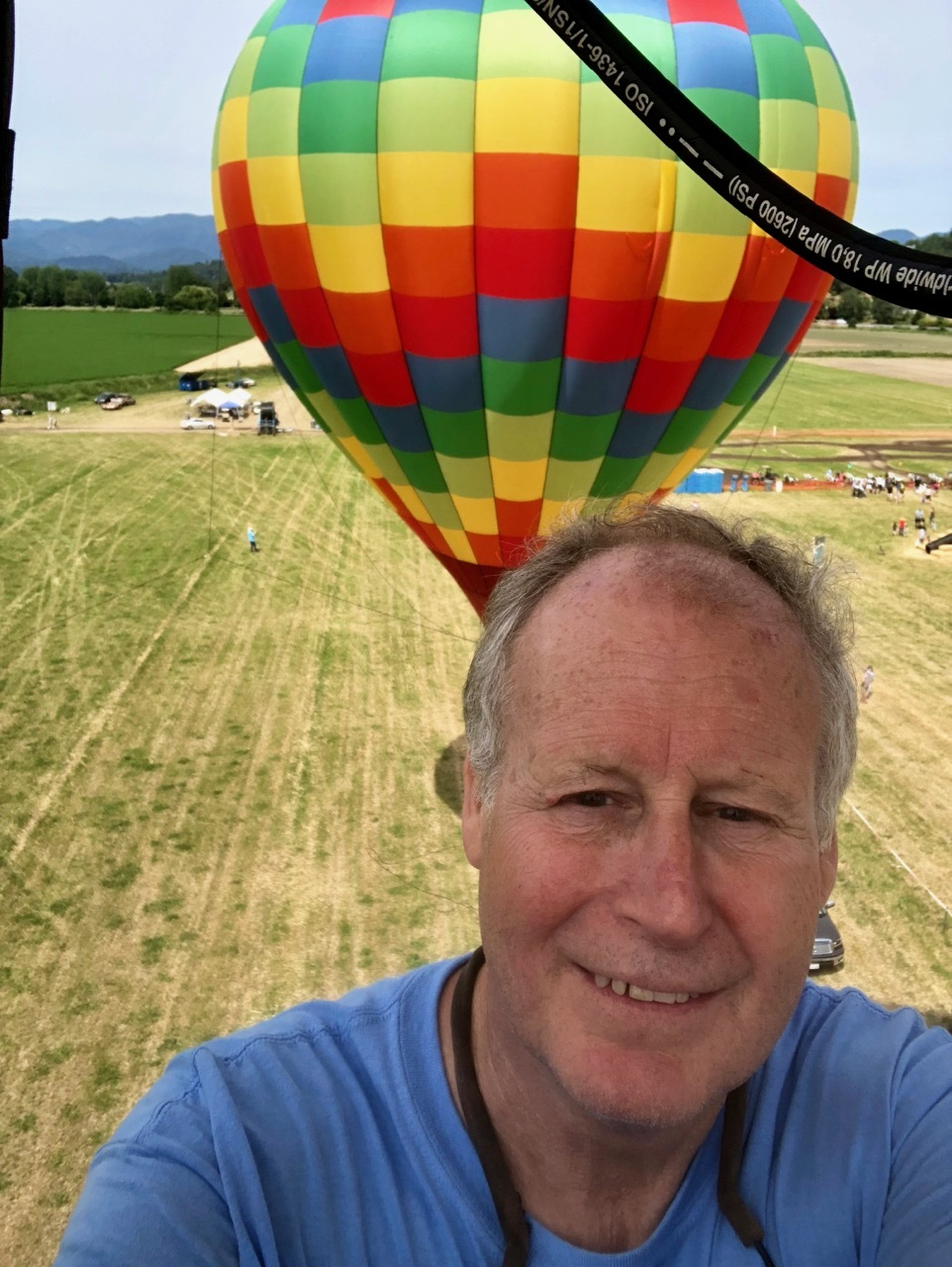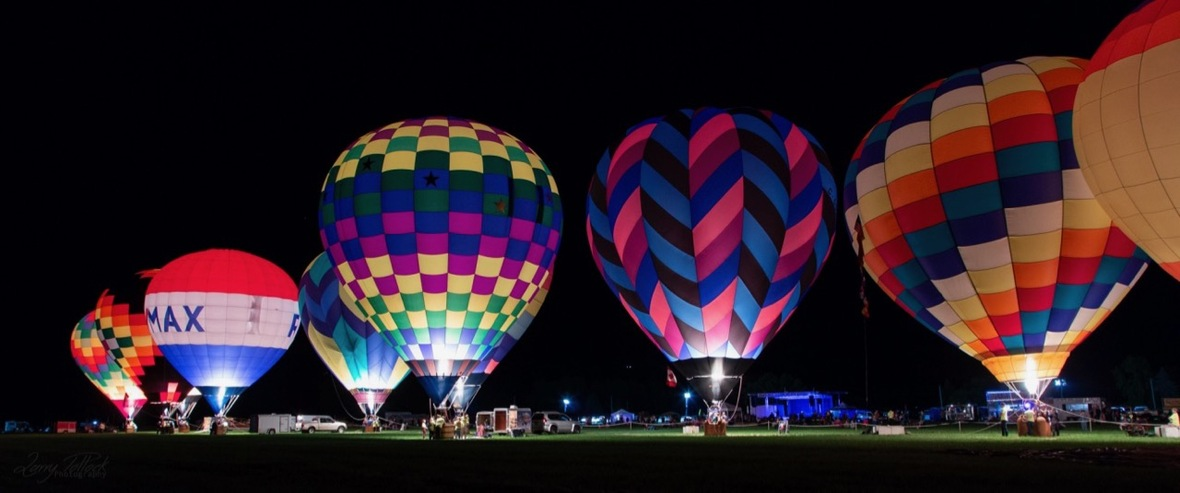table div table+table+table+table+table+table+table+table+table+table+table+table+table+table+table+table+table+table+table+table+table+table+table+table div table{width:100%;padding:0}table div table+table+table+table+table+table+table+table+table+table+table+table+table+table+table+table+table+table+table+table+table+table+table+table div table img{width:96.23%;padding:0;float:none}table div table+table+table+table+table+table+table+table+table+table+table+table+table+table+table+table+table+table+table+table+table+table+table+table div table td{width:100%;padding:0 1.88% 18px}/* styles */